Take full advantage of logical functions on Google Sheets, including NOT, AND, OR, etc. Learn how to combine them with the IF function and comparison operators.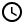1. The Logical Functions: NOT(), AND(), OR()

2. Combining Logical Functions with Comparison Operators

1. Checking whether both of the two requirements are satisfied
2. Checking whether at least one of the two requirements are satisfied

3. Combining Logical Functions with Conditional Formulas

1. Using IF() function
2. Using IFS() function

4. A Sample Sheet to Study

If you have been using Google Sheets for some time, you must be familiar with some of its features such as the Pivot Table, the Slicer, and Conditional Formatting. Today, we will tackle the logical operators in Google Sheets.

If you tried mastering the basics of computer programming, logical operators are considered foundational knowledge and are included in every crash course in programming.

Logical operators are fundamental because they allow computers to do rudimentary decision-making. For example, even a relatively simple process of sorting orders by geographical region requires logical operators to sort them correctly.

## The Logical Functions: NOT(), AND(), OR()

Logical operators test a certain set of conditions, triggering a certain event once those set of conditions are met (or not met). There are three basic logical operators: the NOT, the OR, and the AND operator. Google Sheets implement them as NOT(), OR(), and AND() functions, respectively.

The NOT() function functions like the NOT logical operator: it flips the value from TRUE to FALSE or from FALSE to TRUE.

The AND() function checks two cells if both are TRUE or not; if both cells are TRUE, then the AND() function outputs a TRUE value; else the output is FALSE.

The OR() function outputs a TRUE value if at least one of two cells it references hold a TRUE value; if both cells contain FALSE value, then its output is FALSE.

The table below summarizes the results of the listed logical functions above given the various combinations of TRUE and FALSE values.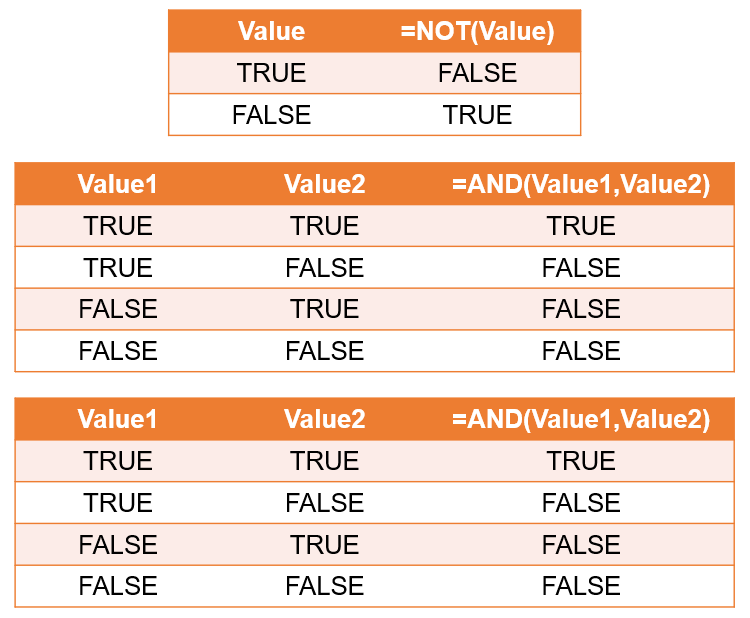Table 1. The table of values from combinations of two TRUE or FALSE values as evaluated by the NOT(), AND(), and OR() functions.

While the NOT() function only works with a single cell as a reference, both AND() and OR() functions also work even if the input comes from more than two cells. The same rules apply:

• The AND() function will output a FALSE value if at least one of the cells in the reference array contains a FALSE value.
• The OR() function will output a TRUE value if at least one of the cells in the reference array contains a TRUE value.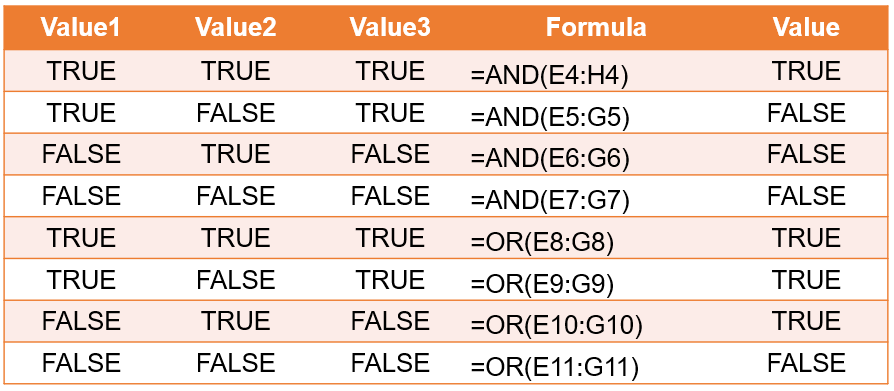Table 2. The table of values from combinations of three TRUE or FALSE values as evaluated by the AND(), and OR() functions. The reference called by the functions is in the form of an array.

## Combining Logical Functions with Comparison Operators

To further appreciate the power of logical functions, we will try combining them with comparison operators, which are used to compare two values and check if a certain condition is fulfilled.

Comparison operators include the equal to operator, the greater than operator, and the less than operator. The table below lists the comparison operators, their corresponding symbols, and the equivalent functions.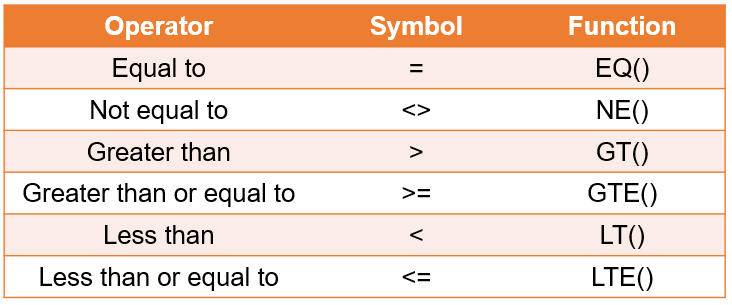Table 3. The table of operators with their corresponding symbols and functions.

The comparison operators, when used as part of a formula, outputs either a TRUE or FALSE value. This behavior allows comparison operators to be combined well with the logical functions. For example, we want to compare two numbers on different cells. To use the comparison operator, we simply follow the following format:

=<cell1><comparison_operator_to_use><cell2></cell2></comparison_operator_to_use></cell1>

Or, when using the comparison functions:

=<cell1><comparison_operator_to_use><cell2></cell2></comparison_operator_to_use></cell1>

The table below shows how the comparison operators work.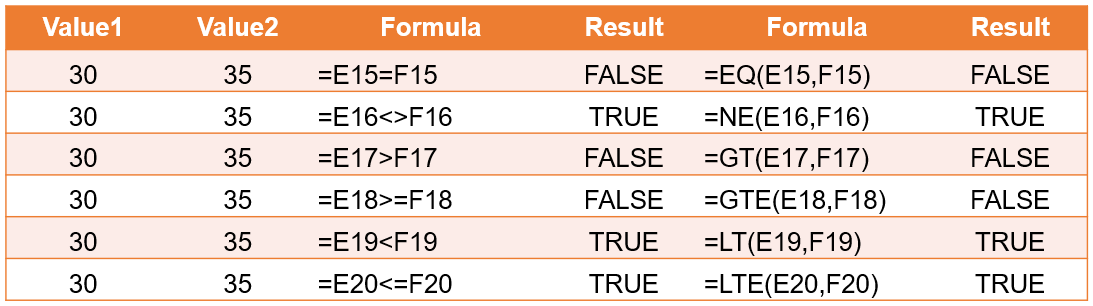Table 4. The comparison operators and functions used to compare two cells.

The three logical functions also allow formulas containing comparison operators or functions as input. To see how it works, let us have a set of examples combining logical functions with comparison operators.

### Checking whether both of the two requirements are satisfied

The AND() function is useful for finding out if two requirements are satisfied. For example, we want to check if the gross profit exceeds a certain amount and that the total number of orders is less than a certain benchmark amount. To do so, we write the following formula:

=AND(B3>175000,B5

And the result looks like this:

### Checking whether at least one of the two requirements are satisfied

The OR() function is useful for finding out if one of the two requirements are satisfied. For the same example as above, we want to check if either the gross profit exceeds a certain amount or that the total number of orders is less than a certain benchmark amount. To do so, we write the following formula:

=OR(B11>175000,B13

And the result looks like this:

## Combining Logical Functions with Conditional Formulas

We'll complete this tutorial by combining the logical functions with conditional formulas IF() and IFS().

### Using IF() function

We want to spice up our example of checking whether the two requirements are satisfied by coding it such that the cell will display “Benchmark satisfied :)” if both requirements are satisfied; else the cell will display “Benchmark not satisfied :(“. The formula will look like this:

=IF(AND(F3>175000,F5

Note this portion:

AND(F3>175000,F5

This portion is the condition being checked by the IF() function, with its result used to do whatever action corresponds to the resulting value.

The result looks like this:

### Using IFS() function

The IFS() function allows you to check if at least one of the several conditions are satisfied and implement the corresponding formula. We wish to fully upgrade our benchmark-checking sheet by also including a way to check what requirement is not satisfied. To do so, we need to list down what should happen for every possible scenario:

If both requirements are satisfied, print “Benchmarks satisfied :)”. To do so, we use the following condition:

AND(F3>175000=TRUE,F5

If the gross profit requirement is satisfied but the maximum total orders requirement is not satisfied, print “Total order amount exceeds benchmark”. To do so we use the following condition:

AND(F3>175000=TRUE,F5

If the gross profit requirement is not satisfied but the maximum total orders requirement is satisfied, print “Gross profit below benchmark”. To do so we use the following condition:

AND(F3>175000=FALSE,F5

If both the gross profit requirement and the maximum total orders requirement are not satisfied, print “Both benchmarks not satisfied :(“. To do so we use the following condition:

AND(F3>175000=FALSE,F5

We combine them in a single IFS() formula:

=IFS(AND(F11>175000=TRUE,F12<2250=true),"benchmarks satisfied="" :)",and(f11="">175000=TRUE,F12<2250=false),"total order="" amount="" exceeds="" benchmark",and(f11="">175000=FALSE,F12<2250=true),"gross profit="" below="" benchmark",and(f11="">175000=FALSE,F12</2250=true),"gross></2250=false),"total></2250=true),"benchmarks>

And the result looks like this:

## A Sample Sheet to Study

I do hope you learned a lot from this tutorial! To help you further learn, here is a sample sheet where you can check the examples shown here: Logical Operators Sample Sheet.